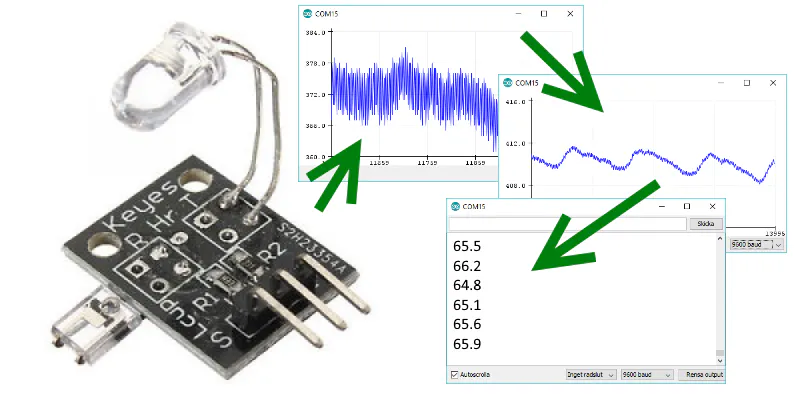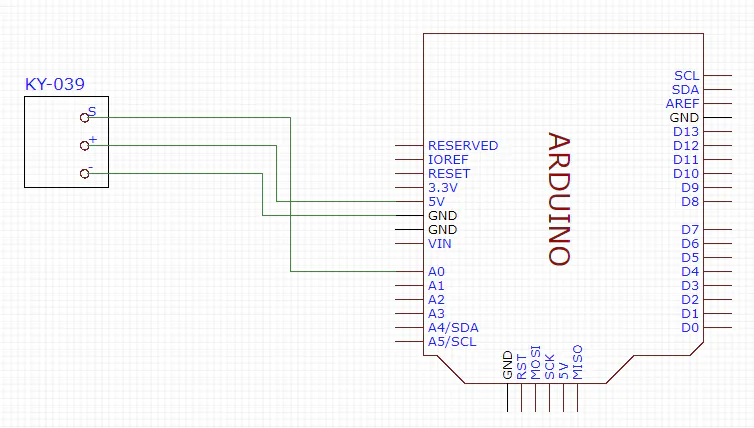# From KY-039 To Heart Rate

BeginnerFull instructions provided23,458## Things used in this project

### Hardware componentsArduino UNO & Genuino UNO
×1
 KY-039 heartbeat sensor
×1Jumper wires (generic)
×1

## Schematics

### Circuit## Code

### Final version

C/C++
The program reads the heartbeat and prints the rate in the serial window.
```#define samp_siz 4
#define rise_threshold 4

// Pulse Monitor Test Script
int sensorPin = 0;

void setup() {
Serial.begin(9600);
}

void loop ()
{
long int now, ptr;
float first, second, third, before, print_value;
bool rising;
int rise_count;
int n;
long int last_beat;

for (int i = 0; i < samp_siz; i++)
sum = 0;
ptr = 0;

while(1)
{
// calculate an average of the sensor
// during a 20 ms period (this will eliminate
// the 50 Hz noise caused by electric light
n = 0;
start = millis();
do
{
n++;
now = millis();
}
while (now < start + 20);
reader /= n;  // we got an average

// and subtract the oldest measurement from the array
// to maintain a sum of last measurements
last = sum / samp_siz;
// now last holds the average of the values in the array

// check for a rising curve (= a heart beat)
if (last > before)
{
rise_count++;
if (!rising && rise_count > rise_threshold)
{
// Ok, we have detected a rising curve, which implies a heartbeat.
// Record the time since last beat, keep track of the two previous
// times (first, second, third) to get a weighed average.
// The rising flag prevents us from detecting the same rise more than once.
rising = true;
first = millis() - last_beat;
last_beat = millis();

// Calculate the weighed average of heartbeat rate
// according to the three last beats
print_value = 60000. / (0.4 * first + 0.3 * second + 0.3 * third);

Serial.print(print_value);
Serial.print('\n');

third = second;
second = first;

}
}
else
{
// Ok, the curve is falling
rising = false;
rise_count = 0;
}
before = last;

ptr++;
ptr %= samp_siz;

}
}

```

## Credits

### Johan_Ha

2 projects • 11 followers